### Go complexity Go forum

55 replies. Last post: 2014-10-19

Go complexity
•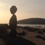Carroll ★ at 2014-10-01

the number of positions after two moves is 129,960…

This seems a gross over estimation not taking into consideration the numbre of symmetries.

After having done a calculation over a blackboard the number I came accross does not give any google hits.

Does anyone here know the real number of different Go positions after two moves? After one move it is 55.

•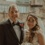wanderer_bot at 2014-10-01

Why wouldn't it just be: 55 * (19^2 - 1) = 19800?

•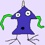EmTom at 2014-10-01

Who cares? Let's just play!

•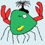William Fraser at 2014-10-01

If black plays on the center point, there are 54 possible white responses.

If black plays one of the remaining 9 points along the main diagonal, there are 18 responses along the same diagonal, plus an additional (361-19)/2 = 171 responses.

Likewise, if black plays one of the 9 points along the center of the board, there are 18 + 171 responses.

Finally, if black plays one of the remaining 36 moves, there are 360 possible responses.

If my envelope matches your blackboard, thats:

54 + 18 * 189 + 36*360 = 16416

And I do not see that in google, either…..

•Carroll ★ at 2014-10-01

Thanks Bill, that is what I got too!

•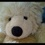richyfourtytwo ★ at 2014-10-01

If you compute one or two more terms you should submit the result to OEIS.

•Carroll ★ at 2014-10-03

I got a new term for 3-plies games: 5834862 which is only 355.4375 times the number of 2-plies games (which it confirmed).

But the program I used is too memory greedy so won't give a fourth term, anyone with an idea of a memory efficient algorithm?

I generated all the games with 2 moves, and the associated 8 symmetries positions, counting only the games yielding new positions, and for these ones generating all the 3 moves games, again counting only the ones yielding new positions…

•Carroll ★ at 2014-10-03

Well I must have a bug, so 3-plies figure wrong. Working on it…

•Carroll ★ at 2014-10-03

I sorted it out. I had made a confusion between the number of different 3-plies games and the number of different positions you achieve. But I counted them well.

For 3-plies games, two games give the same position with Black move order reversed, so to get the number of different positions reached one must divide the number of games by two, hence: 2917431.

A remark from a colleague of mine: for 3-plies games, you don't have to take into account Go rules (even if sometimes you end up with only two stones), but for 4-plies games you have to take into account forbidden suicides in the corners…

•William Fraser at 2014-10-03

You still don't have to take into account the rules if you are just counting positions (because you can assume that the captured stone was the first move by that player).

•richyfourtytwo ★ at 2014-10-04

Hi Caroll,

I think I have an algorithm that works with basically no memory. However, I very much distrust it (or at least I distrust my code), because a) I could confirm your 16416 with it, but I got that number already while there were still several severe bugs in the code (I still get the same number after fixing, but who knows how many other bugs are in there) and b) because for 3 moves a get a number different from yours: 2919078

In case it's easy for you to generate, could you post what you get for some smaller odd board sizes (3,5,7,…) for 3 moves?

•richyfourtytwo ★ at 2014-10-04

Hmm, I've thrown out all optimisation now, which made the code pretty complicated and error prone. I'm still getting 2919078 though. So maybe I'm missing something.

This is what I do: Generate all positions with 2 black stones and one white stone in three simple loops, making sure b1>b3, b1!=w and b2!=w. After a position is generated, generate all 7 symmetric positions. For each position calculate a unique ID. Count the position only if the original positions ID was the minimum in the symmetry set. That way in each symmetry set only one position is ever found. This is pretty slow, as I need to generate everything and also need to generate symmetry transformations for all that, but I don't need to save any positions, so the algorithm needs nearly no memory. (Execution time (unoptimised) for 3 stones was about 5 minutes. 4 stones might be around 20 hours, but I'm not going to try that unless I'm sure my algorithm is correct.)

Any idea what is wrong with my approach / why I'm getting a number different from yours?

•wanderer_bot at 2014-10-04

I tried to do it analytically, ala Bill, and came up with a third result! If anyone is willing to slog through this, maybe they'll see a mistake.

I focused on the different places the white stone could be.

1. The white stone is on the center point.

(a) If one of black's stones is on one of the symmetric lines (diagonal or middle) then the other black stone could be (i) on the same line and on the same half: (9 C 2) = 36 or (ii) on the same line and the other half: 9 x 9 = 81 or (iii) on one side of the symmetric line: 9 x (361 - 19 / 2) = 1539. (1539 + 81 + 36) x 2 = 1656

(b) Both of blacks's stones are in the same symmetric region but not on a boundary line: (36 C 2) = 630

(c) One black stone is in this region and the other is not and the two are not symmetrical counterparts: 36 x (361 - 36 - 73) / 2 where I am subtracting 36 because it is not in the same region, subtracting 73 for all points on symmetric lines since they have already been counted in (a), and dividing by 2 since each pair is actually counted twice. = 4536

(d) Same as (c) but the two black stones occupy symmetrically equivalent points: 36

36 + 4536 + 630 + 1656 = 6858

2. The white stone is on one of the 9 + 9 = 18 points on a symmetric line.

(a) Both black stones are in the same half of the symmetric line, including the line. There are 189 such points (white is taking up one of them) so (189 C 2) = 17766.

(b) One black stone on one half, the other stone on the other half but not symmetrical: (171 x 170) / 2  = 14535  (Again, dividing by 2 since each position will be counted twice.)

(c) One stone in each half and symmetric with respect to the white stone: 171

18 x (17766 + 14535 + 171) = 584496

3. The white stone is one of the other 36 points. In this case we can just place the two black stones: 36 x (360 C 2) = 2326320

Total: 2326320 + 584496 + 6858 = 2917674

•Carroll ★ at 2014-10-04

I launched my program for n=5 and 7and horror the result was odd…

So I got back to my envelop for an analytical solution and came up with the formula for 2-plies: n(n³+3n-4)/8 which gives the values 12, 85, 315 for boards of size 3, 5, 7 and the right 16416 for n=19…So I went one step further and got the 3-plies formula n(n⁵-3n³+4n²-10n+8)/8 which gives the values 66, 1755, 13923 for boards of size 3, 5, 7 and… for size 19, to my surprise 5834862like my program.

But I still don't understand why this should not be divided by two !?

•Carroll ★ at 2014-10-04

@wanderer (or Richard?)I spotted some positions that are counted twice in 2)(a) or (b): if the first black stone is on the same axis as the white stone, not all the 10x19 half plane should be taken,but only 10x9 as putting a black stone on the other 10x9 is the same position, for example white at 10,1 black1 at 10,2 ; then a black2 at 1,1 is the same as at 19,1.Also I don't get where 171 comes from.In (c) where you don't divide by two, I wonder if it is the solution to my problem of getting odd numbers.

•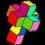ypercube ★ at 2014-10-04

My formula for positions after 3-plies (which could be wrong) gives for sizes 3,5,7,…,19:

38, 898, 7008, 32204, 108370, 296958, 703388, 1494328, 2917854

•William Fraser at 2014-10-05

For size 3, I getWhite center:  6 blackWhite edge:  13 blackWhite corner:  13 black

32

Thoughts?

•William Fraser at 2014-10-05

Oops.  I missed 6.  I now agree with ypercube's 38.

•richyfourtytwo ★ at 2014-10-05

Here are my results for board sizes 3,5,…,19: 38,904,7038,32288,108550,297288,703934,1495168,2919078

Seems we have a few votes for f(3,3) = 38. (A shame that math isn't democratic!)

The differences between ypercube's results and mine are: 0,6,30,84,180,330,546,840,1224

The formula for those differences obviously is (n3-6n2+11n-6)/4.

•richyfourtytwo ★ at 2014-10-05

@wanderer (or Richard?)

I also tried it analytically now and I get the same results as you for 2. and 3. I've been using somewhat different methods for 2.

I'll have a closer look at 1. later we our result differ.

Needless to say that my current total differs from the output of my code and any other result posted so far!

•richyfourtytwo ★ at 2014-10-05

I found a few errors in my calculation of 1), but I still get a result different from yours: 6714

Again the total result differs from any other so far: 2917530

Sigh!

•richyfourtytwo ★ at 2014-10-05

I've changed my code so it now also gives results for 1), 2) and 3) individually. The results for 2) and 3) again agree with our analytical results (wanderer's and mine). So I'm starting to get confident about those parts.

The result for 1) (white point in center) it gives is 8262.

•wanderer_bot at 2014-10-05

@Carroll: You said: “for example white at 10,1 black1 at 10,2 ; then a black2 at 1,1 is the same as at 19,1.” (1, 1) and (19, 1) are on different different sides of the axis of symmetry, so only 1 of them is counted. I think we're OK there.

The 171 in 2 (c) completes the count that is left unfinished in 2 (b). If black has a stone on each half of the axis of symmetry those two stones could be symmetrically placed (that's the 171 in (c)) or they are not, which is what (b) counts.

@richyfortytwo: I found mistakes in my 1) as well. It is more complicated than I realized! One obvious mistake: (1539 + 81 + 36) x 2 = 3312, not 1656, so I need to add 1656. But (b) and (c) are tricky. I obviously forgot to subtract off the symmetric stones in (b) so I think 36 x (361 – 36 – 73) / 2 should be 36 x (361 – 36 – 73 – 3) / 2 = 4482, meaning I need to subtract 54. Similarly, instead of adding 36 back for the symmetric points, there are actually 36 x 3 symmetric points, so I should add 108, meaning I was 72 short. (Though I have to confess I'm still not 100% confident in this analysis!)

So, assuming some of this is right :), I need to correct my previous number for part 1) by +1656 - 54 + 72 = 1674.

Thus, my current calculation for 1) is 6658 + 1674 = 8332 which, of course, is different from yours.

-Richard (who was too lazy to switch accounts on the first post and is now committed to staying with this handle throughout this thread)

•wanderer_bot at 2014-10-05

P.S. Sorry about the bad formatting. I'd become a member again in an instant if we could delete messages.

P.P.S. Wouldn't you think that 1) would be the easiest of the 3 to calculate? Are we missing a simpler approach for that one?

•richyfourtytwo ★ at 2014-10-05

OK, I found many more mistakes and finally my code and my analytical solution give the same result:

8262 + 584496 + 2326320 = 2919078

However, that sounds more convincing than it is. My error correction method was this: Get information from the code calculation about the numbers of the different terms used in the analytical solution. Ignore all that agree and look at the differing ones until you find an error. Repeat. I swear in my correcting I have not been trying to match the 'target number'. But If I were you, I wouldn't believe me! :-)  Also I have been convinced to have the correct solution a few times before. Each time I found new errors on all different levels. So my confidence would still be zero, if not for the agreement of both results. With it I am somewhat optimistic, but far from sure.

BTW, for the first term on board size 5 I get 44. (Size 3 is to special to be interesting.) That might be small enough to compare results by hand. (Of course I have no function yet to return a position in a human readable format, but that shouldn't take too long. Will do it if there is anyone interested in comparing.)

•William Fraser at 2014-10-05

So, trying to get the first term (white stone in center) for board size 5.  I will try by hand:

two black stones on same symmetry half-line:  2two black stones on opposing symmetry half-line:  6two black stones on 90-degree symmetry line:  6two black stones on any other configuration of symmetry line:  8one black stone on a symmetry-line:  16zero black stones on a symmetry-line:  6

44 – matching richyfourtytwo

•William Fraser at 2014-10-05

So, trying to get the first term (white stone in center) for board size 5.  I will try by hand (reposting to get better formatting):

two black stones on same symmetry half-line:  2

two black stones on opposing symmetry half-line:  6

two black stones on 90-degree symmetry line:  6

two black stones on any other configuration of symmetry line:  8

one black stone on a symmetry-line:  16

zero black stones on a symmetry-line:  6

44 – matching richyfourtytwo

•richyfourtytwo ★ at 2014-10-05

Interesting William, your way of separating the different cases is exactly what I used. My last mistake and the hardest to find was the very last term: zero black stones on a symmetry-line:  6 (I had 7 for that before.)

•William Fraser at 2014-10-05

@wanderer_c, In part of your calculation:  36 x (361 – 36 – 73 – 3) / 2  You are dividing an odd number by two, so I think you must have made an error somewhere….

•ypercube ★ at 2014-10-05

I had an error in my calculations. I now agree with richy42's results (added below for n=3,5,…,19,21,23 and 37):

38, 904, 7038, 32288, 108550, 297288, 703934, 1495168, 2919078, 5328200, 9205438, …, 160030728

•Carroll ★ at 2014-10-05

My program agrees with ypercube's figures when I sort the the black stones coordinates not to count them twice.

My previous formula was for three-colors Go, so not very interesting.

Now we just need an analytic formula and we are done, which is not so hard as it must be of degree 6.

Thanks to all for your efforts!

•richyfourtytwo ★ at 2014-10-05

Using http://www.solvemymath.com/online_math_calculator/interpolation.php I get:

(x6-3x4+8x3-13x2+8x-1)/16

I haven't checked though. I have all my individual terms in x, but I'm to lazy to add them all and simplify. At least tonight.

I have started computing the 4th term under the assumption that William was correct with his statement “You still don’t have to take into account the rules if you are just counting positions (because you can assume that the captured stone was the first move by that player).” I think he is. Since the algorithm is stupid and slow and I'm not running it uninterrupted it'll take some time to finish (might be weeks).

•Carroll ★ at 2014-10-05

The formula seems to be :For n=19•Carroll ★ at 2014-10-05

@richyfourtytwoWe crossed posts… and we agree.

Just curious how you will try to compute for four moves?You just add two more loops of size 19, and sort both on black moves and white moves?And yes I think too that Bill is right that the capture position is reachable without conflicting Go rules. Maybe it is worth translating your program to C for performance if you know what hash function to use?

•William Fraser at 2014-10-05

Hi, Caroll.  Your formula isn't showing up on my browser (Safari).

(Just a box with a question mark in it)

•Carroll ★ at 2014-10-06

Yes they were copies of images from Wolfram which are transient. 1/16(n-1)2(n4+2n^3+6n-1)

•richyfourtytwo ★ at 2014-10-06

Hi Caroll,

It's just adding two more loops really. The symmetry check works independently from the number of stones. The algorithm is dumb, but that comes with the advantage of being easy to expand.

How do you know I'm not using C? Well, you are right, I'm not, I'm using java. It's ages since I last worked in C++. If you want I can send you my code. I think I still have your email address, unless it has changed since 2009. Just let me know. (But be prepared to find crappy code.)

It seems to be a bit quicker than anticipated btw, I might get the result in a couple of days. So it might not really be worth the trouble to port it. OTOH we could think about 5 moves. That might be doable with a somewhat quicker program (C?) and distributing the load. However, the assumption that go rules do not need to be considered is wrong for 5 moves unfortunately:

Black: a1, c1, b2

White: b1, a2

Here you cannot tell what the position should be, as it depends on the order of play. It should be counted as 2 positions.

Also the following position can be reached in 5 moves:

Black: a1, b1, a2

White: one stone anywhere else

It might be possible to list all such cases and apply a correction to the number gotten by the naive algorithm.

•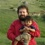Maurizio De Leo at 2014-10-06

Perft for go.

•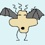gamesorry at 2014-10-07

I try to derive the formula for the first term (with white in the center) on board size n. For convenience let n = 2k + 1, i.e. k is the width of the half-board (except the center lines).

Class A:4 rays on the symmetry-lines x=0 and y=0: x+, x-, y+, y-, containing k stones each.

Class B: 4 rays on the symmetry-lines x=y and x=-y: x+y+, x-y+, x-y-, x+y-, containing k stones each.

(Class A and B could be merged)

Class C: The 8 regions between neighboring Class A and Class B, containing p := (1 + (k-1))*(k-1)/2 = k(k-1)/2 = C(k, 2) stones each.

Then, for the two black stones:

1) AA (same ray): C(k, 2)

2) A*A (different rays): C(k, 2) + k, with 2 patterns (90 and 180 degrees rotation)

3) BB (same ray): C(k, 2)

4) B*B (different rays): C(k, 2) + k, with 2 patterns (90 and 180 degrees rotation)

5) CC (same region): C(p, 2)

6) C*C (different regions): C(p, 2) + p, with 6 patterns (90 and 180 degrees rotation and 4 reflections with 4 symmetry axes)

7) A*B: k * k, with 2 patterns (45 and 135 degrees)

8) A*C: k * p, with 4 patterns (0, 45, 90, 135 degrees)

9) B*C: k * p, with 4 patterns (0, 45, 90, 135 degrees)

We can see that A and B are essentially equivalent, so if we merge A and B, we might get similar representations as William and richyfourtytwo.

Therefore, the final result would be:

C(k, 2) + (C(k, 2)  + k) * 2 + C(k, 2) + (C(k, 2) + k) * 2 + C(p, 2) + (C(p, 2) + p) * 6 + k * k * 2 + k * p * 4 + k * p * 4

= p + (p + k) * 2 + p + (p + k) * 2 + C(p, 2) + (C(p, 2) + p) * 6 + k * k * 2 + k * p * 4 + k * p * 4

= 6p + 4k + p(p-1)/2 + p(p+1)/2 * 6 + 2k^2 + 8kp

Here are examples of the computation for thefirst term (with white in the center)

n = 3, ans = 6 = 0 + 2 + 0 + 2 + 0 + 0 + 2 + 0 + 0

n = 5, ans = 44 = 1 + 6 + 1 + 6 + 0 + 6 + 8 + 8 + 8 (matching withresults from William and richyfourtytwo)

n = 7, ans = 159 = 3 + 12 + 3 + 12 + 3 + 36 + 18 + 36 + 36

n = 9, ans = 417 = 6 + 20 + 6 + 20 + 15 + 126 + 32 + 96 + 96

n = 11, ans = 905 = 10 + 30 + 10 + 30 + 45 + 330 + 50 + 200 + 200

n = 13, ans = 1731 = 15 + 42 + 15 + 42 + 105 + 720 + 72 + 360 + 360

n = 15, ans = 3024 = 21 + 56 + 21 + 56 + 210 + 1386 + 98 + 588 + 588

n = 17, ans = 4934 = 28 + 72 + 28 + 72 + 378 + 2436 + 128 + 896 + 896

n = 19, ans = 7632 = 36 + 90 + 36 + 90 + 630 + 3996 + 162 + 1296 + 1296

n = 21, ans = 11310 = 45 + 110 + 45 + 110 + 990 + 6210 + 200 + 1800 + 1800

n = 23, ans = 16181 = 55 + 132 + 55 + 132 + 1485 + 9240 + 242 + 2420 + 2420

n = 25, ans = 22479 = 66 + 156 + 66 + 156 + 2145 + 13266 + 288 + 3168 + 3168

n = 27, ans = 30459 = 78 + 182 + 78 + 182 + 3003 + 18486 + 338 + 4056 + 4056

n = 29, ans = 40397 = 91 + 210 + 91 + 210 + 4095 + 25116 + 392 + 5096 + 5096

n = 31, ans = 52590 = 105 + 240 + 105 + 240 + 5460 + 33390 + 450 + 6300 + 6300

n = 33, ans = 67356 = 120 + 272 + 120 + 272 + 7140 + 43560 + 512 + 7680 + 7680

n = 35, ans = 85034 = 136 + 306 + 136 + 306 + 9180 + 55896 + 578 + 9248 + 9248

n = 37, ans = 105984 = 153 + 342 + 153 + 342 + 11628 + 70686 + 648 + 11016 + 11016

•gamesorry at 2014-10-07

For the second term (the white stone is on the symmetric line but not the center)on board size n = 2k + 1, there are two classes:

Class A: the symmetric line, containing 2k slots;

Class B: the two half-board regions, containing nk slots each.

Then, for the two black stones:

1) AA: C(2k, 2)

2) BB (same region): C(nk, 2)

3) B*B (different regions): C(nk, 2) + nk

3) AB: 2k * nk

The white stone could be placed at 2k different locations. Therefore, the final result for the second term would be

2k * (C(2k, 2) + C(nk, 2) + C(nk, 2) + nk + 2k * nk)

=2k * (k(2k-1) + nk(nk-1) + nk + 2nk^2)

=2k * ((nk)2 + 2nk2 + 2k^2 - k)

=2k * ((n2 + 2n + 2)k2 - k)

Here are examples for computation for the second term:

n = 3, ans = 32 = 2 * (1 + 3 + 6 + 6)

n = 5, ans = 584 = 4 * (6 + 45 + 55 + 40)

n = 7, ans = 3492 = 6 * (15 + 210 + 231 + 126)

n = 9, ans = 12896 = 8 * (28 + 630 + 666 + 288)

n = 11, ans = 36200 = 10 * (45 + 1485 + 1540 + 550)

n = 13, ans = 85032 = 12 * (66 + 3003 + 3081 + 936)

n = 15, ans = 176204 = 14 * (91 + 5460 + 5565 + 1470)

n = 17, ans = 332672 = 16 * (120 + 9180 + 9316 + 2176)

n = 19, ans = 584496 = 18 * (153 + 14535 + 14706 + 3078) (matching wanderer_c's computation)

n = 21, ans = 969800 = 20 * (190 + 21945 + 22155 + 4200)

n = 23, ans = 1535732 = 22 * (231 + 31878 + 32131 + 5566)

n = 25, ans = 2339424 = 24 * (276 + 44850 + 45150 + 7200)

n = 27, ans = 3448952 = 26 * (325 + 61425 + 61776 + 9126)

n = 29, ans = 4944296 = 28 * (378 + 82215 + 82621 + 11368)

n = 31, ans = 6918300 = 30 * (435 + 107880 + 108345 + 13950)

n = 33, ans = 9477632 = 32 * (496 + 139128 + 139656 + 16896)

n = 35, ans = 12743744 = 34 * (561 + 176715 + 177310 + 20230)

n = 37, ans = 16853832 = 36 * (630 + 221445 + 222111 + 23976)

•gamesorry at 2014-10-07

For the third term (white is not on the axes) on board n = 2k + 1,

positions for white: p = (1 + (k-1))*(k-1)/2 = k(k-1)/2

positions for two black stones: C(n2-1, 2) = (n2 - 1)(n^2 - 2)/2

The final result for the third term would be:

k(k-1)/2 * (n2 - 1)(n2 - 2)/2

Here are examples for computation for the third term:

n = 3, ans = 0 = 0 * 28

n = 5, ans = 276 = 1 * 276

n = 7, ans = 3384 = 3 * 1128

n = 9, ans = 18960 = 6 * 3160

n = 11, ans = 71400 = 10 * 7140

n = 13, ans = 210420 = 15 * 14028

n = 15, ans = 524496 = 21 * 24976

n = 17, ans = 1157184 = 28 * 41328

n = 19, ans = 2326320 = 36 * 64620 (matching wanderer_c's result)

n = 21, ans = 4346100 = 45 * 96580

n = 23, ans = 7652040 = 55 * 139128

n = 25, ans = 12828816 = 66 * 194376

n = 27, ans = 20640984 = 78 * 264628

n = 29, ans = 32066580 = 91 * 352380

n = 31, ans = 48333600 = 105 * 460320

n = 33, ans = 70959360 = 120 * 591328

n = 35, ans = 101792736 = 136 * 748476

n = 37, ans = 143059284 = 153 * 935028

•gamesorry at 2014-10-07

Summing up all three terms, we have:

n = 3, ans = 38 = 6 + 32 + 0

n = 5, ans = 904 = 44 + 584 + 276

n = 7, ans = 7035 = 159 + 3492 + 3384

n = 9, ans = 32273 = 417 + 12896 + 18960

n = 11, ans = 108505 = 905 + 36200 + 71400

n = 13, ans = 297183 = 1731 + 85032 + 210420

n = 15, ans = 703724 = 3024 + 176204 + 524496

n = 17, ans = 1494790 = 4934 + 332672 + 1157184

n = 19, ans = 2918448 = 7632 + 584496 + 2326320

n = 21, ans = 5327210 = 11310 + 969800 + 4346100

n = 23, ans = 9203953 = 16181 + 1535732 + 7652040

n = 25, ans = 15190719 = 22479 + 2339424 + 12828816

n = 27, ans = 24120395 = 30459 + 3448952 + 20640984

n = 29, ans = 37051273 = 40397 + 4944296 + 32066580

n = 31, ans = 55304490 = 52590 + 6918300 + 48333600

n = 33, ans = 80504348 = 67356 + 9477632 + 70959360

n = 35, ans = 114621514 = 85034 + 12743744 + 101792736

n = 37, ans = 160019100 = 105984 + 16853832 + 143059284

•gamesorry at 2014-10-07

Oh, I think I made a mistake in the estimation of the first term in case #6:

6) C*C (different regions): C(p, 2) + p, with 6 patterns (90 and 180 degrees rotation and 4 reflections with 4 symmetry axes)

Actually this should be divided into two cases, i.e,.

6) C*C (different regions):

6a) C(p, 2) + p, with 5 patterns (180 degrees rotation and 4 reflections with 4 symmetry axes)

6b) p * p, with 1 pattern (90 degrees rotation)

The corrected formula for the first term:

C(k, 2) + (C(k, 2)  + k) * 2 + C(k, 2) + (C(k, 2) + k) * 2 + C(p, 2) + (C(p, 2) + p) * 5 + p * p + k * k * 2 + k * p * 4 + k * p * 4

= p + (p + k) * 2 + p + (p + k) * 2 + C(p, 2) + (C(p, 2) + p) * 5 + p * p + k * k * 2 + k * p * 4 + k * p * 4

= 6p + 4k + p(p-1)/2 + p(p+1)/2 * 5 + p2 + 2k2 + 8kp

The computation for the first term:

n = 3, ans = 6 = 0 + 2 + 0 + 2 + 0 + 0 + 2 + 0 + 0

n = 5, ans = 44 = 1 + 6 + 1 + 6 + 0 + 6 + 8 + 8 + 8

n = 7, ans = 162 = 3 + 12 + 3 + 12 + 3 + 39 + 18 + 36 + 36

n = 9, ans = 432 = 6 + 20 + 6 + 20 + 15 + 141 + 32 + 96 + 96

n = 11, ans = 950 = 10 + 30 + 10 + 30 + 45 + 375 + 50 + 200 + 200

n = 13, ans = 1836 = 15 + 42 + 15 + 42 + 105 + 825 + 72 + 360 + 360

n = 15, ans = 3234 = 21 + 56 + 21 + 56 + 210 + 1596 + 98 + 588 + 588

n = 17, ans = 5312 = 28 + 72 + 28 + 72 + 378 + 2814 + 128 + 896 + 896

n = 19, ans = 8262 = 36 + 90 + 36 + 90 + 630 + 4626 + 162 + 1296 + 1296

n = 21, ans = 12300 = 45 + 110 + 45 + 110 + 990 + 7200 + 200 + 1800 + 1800

n = 23, ans = 17666 = 55 + 132 + 55 + 132 + 1485 + 10725 + 242 + 2420 + 2420

n = 25, ans = 24624 = 66 + 156 + 66 + 156 + 2145 + 15411 + 288 + 3168 + 3168

n = 27, ans = 33462 = 78 + 182 + 78 + 182 + 3003 + 21489 + 338 + 4056 + 4056

n = 29, ans = 44492 = 91 + 210 + 91 + 210 + 4095 + 29211 + 392 + 5096 + 5096

n = 31, ans = 58050 = 105 + 240 + 105 + 240 + 5460 + 38850 + 450 + 6300 + 6300

n = 33, ans = 74496 = 120 + 272 + 120 + 272 + 7140 + 50700 + 512 + 7680 + 7680

n = 35, ans = 94214 = 136 + 306 + 136 + 306 + 9180 + 65076 + 578 + 9248 + 9248

n = 37, ans = 117612 = 153 + 342 + 153 + 342 + 11628 + 82314 + 648 + 11016 + 11016

•gamesorry at 2014-10-07

The corrected result for summing up all three terms (matching ypercube's results):

n = 3, ans = 38 = 6 + 32 + 0

n = 5, ans = 904 = 44 + 584 + 276

n = 7, ans = 7038 = 162 + 3492 + 3384

n = 9, ans = 32288 = 432 + 12896 + 18960

n = 11, ans = 108550 = 950 + 36200 + 71400

n = 13, ans = 297288 = 1836 + 85032 + 210420

n = 15, ans = 703934 = 3234 + 176204 + 524496

n = 17, ans = 1495168 = 5312 + 332672 + 1157184

n = 19, ans = 2919078 = 8262 + 584496 + 2326320

n = 21, ans = 5328200 = 12300 + 969800 + 4346100

n = 23, ans = 9205438 = 17666 + 1535732 + 7652040

n = 25, ans = 15192864 = 24624 + 2339424 + 12828816

n = 27, ans = 24123398 = 33462 + 3448952 + 20640984

n = 29, ans = 37055368 = 44492 + 4944296 + 32066580

n = 31, ans = 55309950 = 58050 + 6918300 + 48333600

n = 33, ans = 80511488 = 74496 + 9477632 + 70959360

n = 35, ans = 114630694 = 94214 + 12743744 + 101792736

n = 37, ans = 160030728 = 117612 + 16853832 + 143059284

•wanderer_bot at 2014-10-07

Very nicely done, gamesorry. You've given me something to chew on when I find some free time.

I had to laugh when you said: “The corrected formula for the first term: …“. That first term is surprisingly hard to analyze, isn't it?!

•richyfourtytwo ★ at 2014-10-07

Here's my result for four moves: 522019404

Anyone keen to try that one analytically? :-)

So we now have 55, 16416, 2919078 , 522019404 If nobody else does I'll prepare something for submitting it to OEIS.

Any reason why all terms but the first are even? (Well, with just three terms it's a bit early to suspect a pattern, I admit.)

•William Fraser at 2014-10-07

I'll take a crack at an analytical solution, but not until the weekend.

•richyfourtytwo ★ at 2014-10-07

Of course all our results are wrong, because pass is a legal move too!

•ypercube ★ at 2014-10-07

The sequence should be: 1, 55, 16416, …

•richyfourtytwo ★ at 2014-10-08

OK, lets have a look at what happens if we also allow passing.

Without passing we have so far:

For odd n>=3:

T(n,0) = 1 (Thanks for pointing this out, ypercube!)

T(n,1) = (n+1)(n+3)/8

T(n,2) = n(n^3+3n-4)/8

T(n,3) = (1/16)(n-1)2(n4+2n^3+6n-1)

and also

T(19,4) = 522019404

With passing:

T(n,1) = (n+1)(n+3)/8 + b_passes

T(19,1) = 55

T(n,2) = n(n^3+3n-4)/8  + b_passes + w_passes + both_pass

= n(n^3+3n-4)/8  + (n+1)(n+3)/8 + (n+1)(n+3)/8 + 1

= (n(n^3+3n-4)+ 2(n+1)(n+3) + 8)/8

T(19,2) = 16416 + 111 = 16527

T(n,3) = (1/16)(n-1)2(n4+2n^3+6n-1) + b_passes_once + w_passes_once + b_passes_twice + both_pass_once

both_pass_once = (n+1)(n+3)/8

b_passes_twice = (n+1)(n+3)/8

b_passes_once = n(n^3+3n-4)/8

w_passes_once is the number of positions with two black stones. This fortunately is extremely similar to the number of positions with one white stone in the center and two black stones elsewhere, that we have been discussing ad nauseam. The formula for this seems to be (n4+6n2-16n+9)/16. To this we just have to add the positions with 1 black stone in the center and the other elsewhere, which is just (n+1)(n+3)/8 - 1. So we get:

w_passes_once = (n4+6n2-16n-7+2(n+1)(n+3))/16

So altogether we have

T(n,3) = (1/16)(n-1)2(n4+2n3+6n-1) + n(n3+3n-4)/8 + (n4+6n2-16n-7+2(n+1)(n+3))/16 + (n+1)(n+3)/4

= [(n-1)2(n4+2n3+6n-1) + 2n(n3+3n-4) + (n4+6n2-16n-7+2(n+1)(n+3)) + 4(n+1)(n+3)]/16

= [(n-1)2(n4+2n3+6n-1) + 2n(n3+3n-4) + n4+6n2-16n-7 + 6(n+1)(n+3)]/16

Yeah, this can surely be written more nicely, but I'm too lazy right now.

T(19,3) = 2919078 + 16416 + 8262 + 54 + 110 = 2943920

Does anybody care to check all this?

For the forth move we have no formula, but at least the value for n=19 without passing. I hope getting the correction terms for that one will also be straightforward. I'll try that at some later time if nobody beats me to it.

•gamesorry at 2014-10-09

@wanderer_c Thanks, yeah the first term is harder than I imagined, especially in case #6 which is a little counter-intuitive ;-)

•richyfourtytwo ★ at 2014-10-19

Meanwhile it has occurred to me that the entire thread has ignored superko. If that rule is taken into account the mathematical position of the game in the game tree is not alone defined by the positions of the stones, but also by the positions that lead up to the current positions, as these restrict future legal moves. In that sense I think all numbers we found for 3 or more stones are wrong! :-)

•William Fraser at 2014-10-19

@richyfourtytwo

Wow, what a thought!

However, I think we can safely define the problem either way and this way is definitely more interesting (allowing passing is also interesting, but I would ignore it – after all, there are historical games where someone was forfeited for passing, so it could be considered “illegal”).

•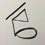hyperpape at 2014-10-19

I believe “position” is used to designate an arrangement of stones, without reference to how it was achieved. E.g: positional superko vs. situational superko. See senseis: http://senseis.xmp.net/?Position, (though the page seems to treat “position” as including some illegal “positions”, which I wouldn't do. Not sure how people who study the game's combinatorial properties talk about that.

•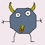fhourplay at 2014-10-19

> though the page seems to treat “position” as including some illegal “positions”, which I wouldn’t do

There are 3^361 positions which can be either legal or illegal. It would be cumbersome to talk

about legal positions versus illegal arrangements, so we equate the notions of arrangement and

position. Every legal position can result from a legal game, that can include passes.